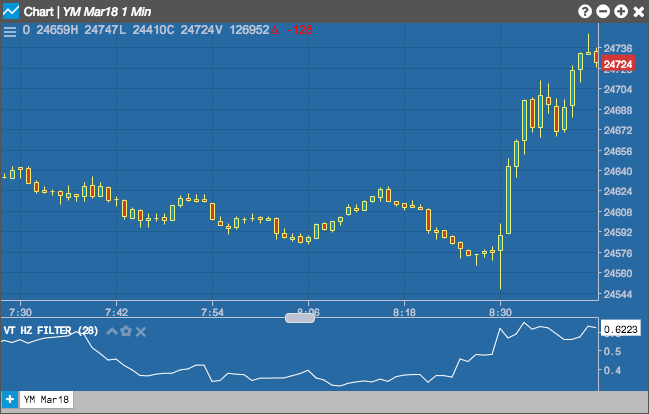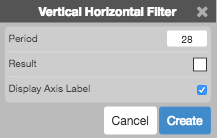Charts

# Vertical Horizontal Filter

Created by Adam White, the Vertical Horizontal Filter helps the user determine whether a particular price is trending. The higher the Vertical Horizontal Filter value, the more a particular contract is trending. In addition, the direction of the Vertical Horizontal Filter shows what phase the Vertical Horizontal Filter is entering:

• A rising Vertical Horizontal Filter means that prices are trending.
• A falling Vertical Horizontal Filter means that prices are stablizing around a specific range.

Selecting the Vertical Horizontal Filter allows you to set the Period (as number of minutes) and the color of the result.## Configuration Options• Period: Number of bars to use in the calculations.
• Color Selectors: Colors to use for graph elements.
• Display Axis Label: Whether to display the most recent value on the Y axis.

## Formula

The Vertical Horizontal Filter is calculated as follows:

$VHF = \frac{ABS(High Closing Price - Low Closing Price)}{ \sum_{j=1}^{n}ABS(Close_{j} - Close_{j-1})}$